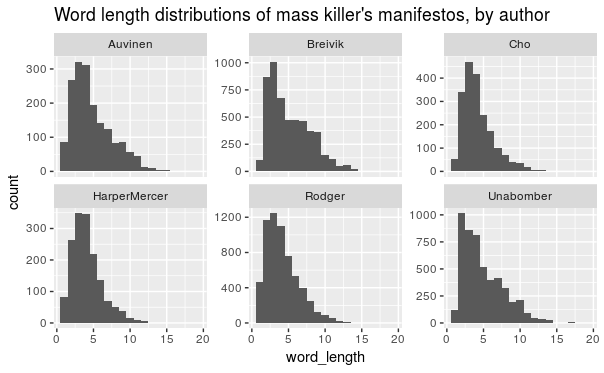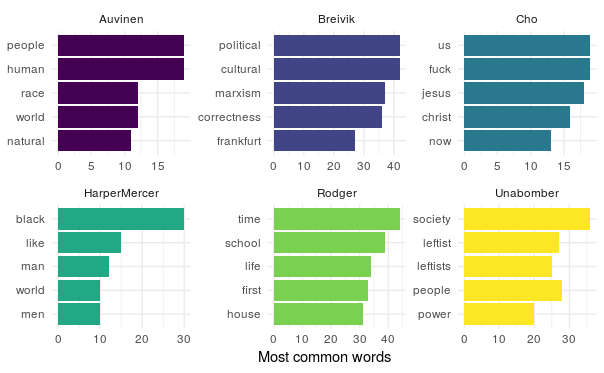``````library(tidyverse)
library(DT)
library(tidytext)

#Question 1

``````killers_notes <- read_excel("manifestos.xlsx")

killers_words <- killers_notes %>%
unnest_tokens(word, text)

killers_words``````

#1 Description: This reads in the set of words used in the mass killer’s manifestos and unnests them so that the words are showed one at a time.

#Question 2

``````killers_words %>%
group_by(author) %>%
summarize(num_words = n(), lex_diversity = n_distinct(word), lex_density = n_distinct(word)/n())``````

#2 Description: This table shows the killer/author’s name, the number of words used in the manifesto, the number of unique words used (diversity), and the proportion of words which were used repeatedly (density).

#Question 3

``````killers_words %>%
group_by(author) %>%
mutate(word_length = nchar(word)) %>%
summarize(mean_word_length = mean(word_length)) %>%
arrange(-mean_word_length)``````

#3 Description: This table shows the killer/author and the average length of their words used in the manifestos.

#Question 4

``````killers_words %>%
mutate(word_length = nchar(word)) %>%
ggplot(aes(word_length)) +
geom_histogram(binwidth = 1) +
facet_wrap(vars(author), scales = "free_y") +
labs(title = "Word length distributions of mass killer's manifestos, by author")``````#4 Description: These graphs show the word lengths distributions by author.

#Question 5

``````
killers_words %>%
anti_join(stop_words) %>%
group_by(author) %>%
count(word, sort = T) %>%
top_n(5) %>%
ungroup() %>%
mutate(word = reorder(word, n)) %>%
ggplot(aes(word, n, fill = author)) +
geom_col(show.legend = FALSE) +
labs(x = NULL, y = "Most common words") +
facet_wrap(vars(author), scales = "free") +
scale_fill_viridis_d() +
theme_minimal() +
coord_flip()``````
``````Joining, by = "word"
Selecting by n``````#5 Description: These graphs show the most common words used in each killer/author’s manifesto.

#Question 6

``````killers_word_counts <- killers_notes %>%
unnest_tokens(word, text) %>%
count(author, word, sort = TRUE)

total_kwords <- killers_word_counts %>%
group_by(author) %>%
summarize(total = sum(n))

killers_word_counts <- left_join(killers_word_counts, total_kwords)``````
``Joining, by = "author"``
``````killers_tf_idf <- killers_word_counts %>%
bind_tf_idf(word, author, n)

killers_tf_idf %>%
arrange(-tf_idf) %>%
anti_join(stop_words)``````
``Joining, by = "word"``
``````killers_tf_idf %>%
anti_join(stop_words) %>%
arrange(-tf_idf) %>%
mutate(word = factor(word, levels = rev(unique(word)))) %>%
group_by(author) %>%
top_n(5) %>%
ggplot(aes(word, tf_idf, fill = author)) +
geom_col(show.legend = FALSE) +
labs(x = NULL, y = "tf-idf") +
facet_wrap(~author, scales = "free") +
coord_flip() +
theme_minimal() +
scale_fill_viridis_d() +
labs(title = "Most distinctive words in each mass killer manifesto")``````
``````Joining, by = "word"
Selecting by tf_idf``````